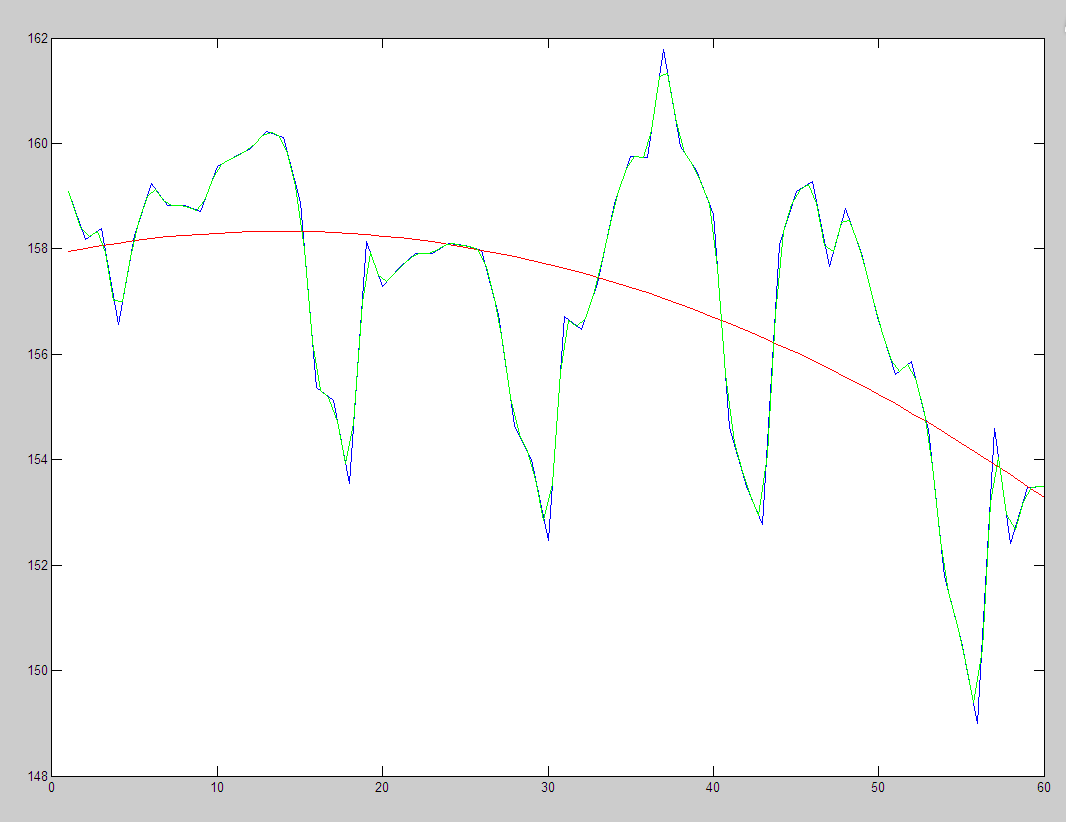• 自然状态下，用plot的是折线，而不是平滑曲线。 有两种方法可以画平滑曲线，第一种是拟合的方法，第二种是用spcrv，其实原理应该都一样就是插值。下面是源程序，大家可以根据需要自行选择，更改拟合的参数。 clc...
自然状态下，用plot画的是折线，而不是平滑曲线。
有两种方法可以画平滑曲线，第一种是拟合的方法，第二种是用spcrv，其实原理应该都一样就是插值。下面是源程序，大家可以根据需要自行选择，更改拟合的参数。
clc,clear; a = 1:1:6;  %横坐标 b = [8.0 9.0 10.0 15.0 35.0 40.0]; %纵坐标 plot(a, b, 'b');   %自然状态的画图效果 hold on; %第一种，画平滑曲线的方法 c = polyfit(a, b, 2);  %进行拟合，c为2次拟合后的系数 d = polyval(c, a, 1);  %拟合后，每一个横坐标对应的值即为d plot(a, d, 'r');       %拟合后的曲线
plot(a, b, '*');       %将每个点 用*画出来 hold on; %第二种，画平滑曲线的方法 values = spcrv([[a(1) a a(end)];[b(1) b b(end)]],3); plot(values(1,:),values(2,:), 'g');
效果：展开全文• x=[0 0.1 0.16 0.27 0.41 0.48 0.59 0.8]; y=[5 9 70 118 100 17 0 5]; y1=[22.8 22.8 22.8 22.8 22.8 22.8 22.8 22.8]; values1=spcrv([[x(1) x x(end)];[y(1) y y(end)]],3,1000); values2=spcrv([[x(1) x x(end)]...
• 自然状态下，用plot的是折线，而不是平滑曲线。 有两种方法可以画平滑曲线，第一种是拟合的方法，第二种是用spcrv，其实原理应该都一样就是插值。下面是源程序，大家可以根据需要自行选择，更改拟合的参数。 clc...
自然状态下，用plot画的是折线，而不是平滑曲线。
有两种方法可以画平滑曲线，第一种是拟合的方法，第二种是用spcrv，其实原理应该都一样就是插值。下面是源程序，大家可以根据需要自行选择，更改拟合的参数。
clc,clear; a = 1:1:6;  %横坐标 b = [8.0 9.0 10.0 15.0 35.0 40.0]; %纵坐标 plot(a, b, 'b');   %自然状态的画图效果 hold on; %第一种，画平滑曲线的方法 c = polyfit(a, b, 2);  %进行拟合，c为2次拟合后的系数 d = polyval(c, a, 1);  %拟合后，每一个横坐标对应的值即为d plot(a, d, 'r');       %拟合后的曲线
plot(a, b, '*');       %将每个点 用*画出来 hold on; %第二种，画平滑曲线的方法 values = spcrv([[a(1) a a(end)];[b(1) b b(end)]],3); plot(values(1,:),values(2,:), 'g');
效果：展开全文• Matlab画平滑曲线的两种方法 拟合或插值后再用plot即可
分享一下我老师大神的人工智能教程！零基础，通俗易懂！http://blog.csdn.net/jiangjunshow

也欢迎大家转载本篇文章。分享知识，造福人民，实现我们中华民族伟大复兴！

自然状态下，用plot画的是折线，而不是平滑曲线。
有两种方法可以画平滑曲线，第一种是拟合的方法，第二种是用spcrv，其实原理应该都一样就是插值。下面是源程序，大家可以根据需要自行选择，更改拟合的参数。
clc,clear;a = 1:1:6;  %横坐标b = [8.0 9.0 10.0 15.0 35.0 40.0]; %纵坐标plot(a, b, 'b');   %自然状态的画图效果hold on;%第一种，画平滑曲线的方法c = polyfit(a, b, 2);  %进行拟合，c为2次拟合后的系数d = polyval(c, a, 1);  %拟合后，每一个横坐标对应的值即为dplot(a, d, 'r');       %拟合后的曲线
plot(a, b, '*');       %将每个点 用*画出来hold on;%第二种，画平滑曲线的方法values = spcrv([[a(1) a a(end)];[b(1) b b(end)]],3);plot(values(1,:),val

展开全文• 自然状态下，用plot的是折线，而不是平滑曲线。 有两种方法可以画平滑曲线，第一种是拟合的方法，第二种是用spcrv，其实原理应该都一样就是插值。下面是源程序，大家可以根据需要自行选择，更改拟合的参数。 clc...
自然状态下，用plot画的是折线，而不是平滑曲线。
有两种方法可以画平滑曲线，第一种是拟合的方法，第二种是用spcrv，其实原理应该都一样就是插值。下面是源程序，大家可以根据需要自行选择，更改拟合的参数。
clc,clear; a = 1:1:6;  %横坐标 b = [8.0 9.0 10.0 15.0 35.0 40.0]; %纵坐标 plot(a, b, 'b');   %自然状态的画图效果 hold on; %第一种，画平滑曲线的方法 c = polyfit(a, b, 2);  %进行拟合，c为2次拟合后的系数 d = polyval(c, a, 1);  %拟合后，每一个横坐标对应的值即为d plot(a, d, 'r');       %拟合后的曲线
plot(a, b, '*');       %将每个点 用*画出来 hold on; %第二种，画平滑曲线的方法 values = spcrv([[a(1) a a(end)];[b(1) b b(end)]],3); plot(values(1,:),values(2,:), 'g');
效果：展开全文• Matlab代码，输入一个Y输出smooth后的Y，适用如下 smoothY = smoothCurve(Y);
• ## Matlab曲线平滑

万次阅读 2019-05-14 22:19:37
semilogy(x1,y1)%原来的折线 x2=linspace(min(x1),max(x1)); y2=interp1(x1,y1,x2,'cubic'); figure semilogy(x2,y2)%处理后的曲线 x=[1,2,3,4,5]; y=[1,4,5,8,12]; plot(x,y);...下面进行曲线平滑： x...
• MATLAB中绘制平滑曲线一般使用最小二乘法或者B样条插值。  最小二乘法实际上是函数拟合，可以得到目标函数（这里为多项式）的系数，对outliers相对不敏感，缺点是需要预先设置目标函数的阶数，且有时不容易找到最优...最小二乘法 B样条
• 1基本命令一般函数或方程式之图形，都是先标上几个图形上的点，进而再将点连接即为函数图形，其点愈多图形越平滑MATLAB在简易2维画图(plot)，也是相同做法，必须先点出x 和y 坐标(离散数据)，再将这些点连接，...
• 曲线拟合曲线拟合可以说在多种领域都有其用武之地，因为其主要的功能就是寻求平滑曲线来更好的表现带有噪声的测量数据。一般对于进行曲线拟合操作，都是先给定一部分采集的离散的数据点，在这些点的基础上建立数学...
• ## matlab绘制平滑曲线

千次阅读 2013-09-27 22:49:09
以上是每一个X和Y对应的坐标，请问如何编程能够绘制平滑曲线，这个图形就像二次函数一样的 如果要在图中绘制一条直线加上y=70的直线，用不同颜色区分！   x=[0 0.1 0.16 0.27 0.41 0.48 0.59 0.8]; y=[5 9 70 ...
• 图像要求矢量图，所以使用MATLAB画图。 其实我内心已经要疯了……被毕设折磨好多天了，自从模型出错之后我整个神经都是绷着的，实验花费的时间又比预计要久，第一天爆肝又让自己生物钟乱掉了，所以，整个人疯了……...
• - PAGE PAGE 3 欢迎下载 MATLAB绘制平滑曲线 x=[0.1 0.16 0.27 0.41 0.48 0.59 0.8]; y=[8 70 118 100 9 0 5]; 以上是每一个X和Y对应的坐标请问如何编程能够绘制平滑曲线这个图形就像二次函数一样的 如果要在图中...
• 需要预先安装matlab curving fitting tool。 直接上代码 x1 = [1.0 1.0 1.2 3.0 5.8 7.2 7.4]; y1 = [283 283 278 215 90 37 0]; x2 = [0.5 0.5 0.5 0.6 1.9 5.8 6.6 6.7]; y2 = [145 145 148 145 145 110 28 0];...
• (3)可视化：数据可以用图像、曲线、二维图形、三维图形和动画等来显示，并可对其模式和相互关系进行可视化分析。 数据可视化可以大大加快数据的处理速度，令时刻都在产生的大量数据得到有效的利用；可以在人与数据...
• 导师要求参照别人论文中的图（下图），将其论文...功能：将散点连接并平滑 1）Matlab 效果图： x1=[431.50032,759.5552,1335.3736,2530.388] %输入以下三组数据 y1=[34.06366,35.73132,37.2244,38.61294] x2=[263.8...数据可视化 python
• 原帖地址：科研画图-率失真曲线图：散点连接并平滑（基于Matlab和Python）_Kobaayyy的博客-CSDN博客_set(gca,fontsize,13,fontweight,bold);python​blog.csdn.net网上关于科研画图相关的代码比较少，就参照别人论文...
• 小编最近写文章的时候发现，训练网络，使用tensorboard进行可视化展示出来的精度曲线下载后生成csv或json类型的文件，小编尝试了使用各种...所以找了一段用matlab进行平滑曲线的程序。挂在上面。总的来说就是~~~~怕丢！
• ![图片说明]...怎样将图片中曲线进行平滑，尤其是对于红色蓝色交界处的平滑，我用 spcrv函数实现有点困难，有没有别的方法，或者spcrv函数使用有没有什么特殊的注意事项，哪位大神指导一下，万分感谢！图片
• Akima 的单变量插值方法的实现（Journal of the ACM，第 17 卷，第 4 期，1970 年 10 月，第 589-602 页）。 N. Shamsundar，休斯顿大学
• Matlab是一种基于数值计算的科技应用和工程研发的软件，全称是Matrix Laboratory(矩阵实验室)。Matlab具有编程简单、直观，友善的用户界面，精美的可视化图形，丰富的数学分析命令，以及众多专业的工具箱等优点。...
• ## MATLAB曲线平滑的办法

万次阅读 多人点赞 2016-05-18 17:41:35
发现了两个MATLAB自带的曲线平滑函数：smooth和spcrv 一、smooth 自己感觉：平滑效果还可以 1.语法 (1)函数 smooth（Y） smooth(Y,SPAN)%SPAN是滑动平均的点数，即“几点滑动平均” smooth(Y,SPAN,METHOD) （2）...
• 曲线拟合也称为曲线逼近，它只要求拟合的曲线合理的反应数据的基本趋势，而并不要求曲线一定经过数据点，只是要求在整体上“尽量好”的逼近原函数。这时,在每个已知点上就会有误差,数据拟合就是从整体...
• 散点连接成曲线出原散点的标记点（基于Matlab）_Kobaayyy的博客-CSDN博客_率失真优化 matlab​blog.csdn.net之前有做过一些科研曲线图的工作，具体见：科研画图：散点连接并平滑（基于Matlab和Python）现在又...
• 通过进行曲线平滑，得到了比较美观光滑的figure 2，于是就可以在论文报告中插入使用啦！ 【matlab代码如下：】 x1=[11.2,11.4,11.6,11.8,12,12.2,12.4,12.6,12.8]; y1=[1.86,2.28,2.34,2.56,2.62,2.04,1.76,1.48......

# matlab画平滑曲线matlab 订阅